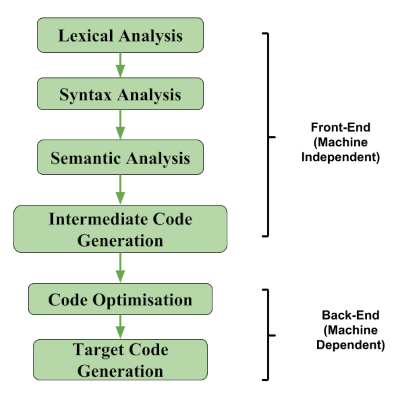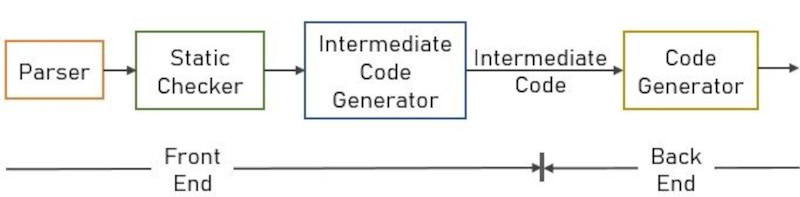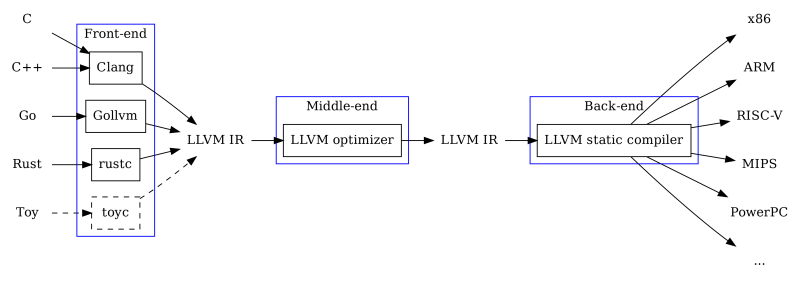×

Search anything:

# Building a Compiler Front-End

#### Compiler DesignThe front-end of a compiler comprises four phases, source code is converted from high-level code to an intermediate representation which is optimized and compiled to machine code.

1. Introduction.
2. The compiler front-end.
3. LLVM.
4. Kaleidoscope.
5. Summary.
6. References.

## Prerequisite.

LLVM: IR, Assembly, SSA.

## The compiler front-end.

The front-end of a compiler comprises four phases, these are lexical analysis, syntax analysis, and semantic analysis.Lexical analysis.
Here source code written in a high-level programming language such as C/C++ is converted into meaningful lexemes which are represented as tokens by the lexer.

Syntax analysis.
The tokens are input in this phase, they are used to produce a parse tree.

Semantic analysis.
Here we check if the parse tree adheres to the semantics of the programming language used.

Intermediate code generation.
IR is code that is between high and low-level machine code. It is readable and at the same time low level.

Functions of the compiler front-end include;

• Determining the validity of source code.
• Determining the content of source code.
• Building source code for easy analysis and optimizations.

The process of compiler design follows the following steps,
First code written in high-level languages such as C/C++ is used as input to the parser which produces an AST(Abstract Syntax Tree) which is the input to e code-generator which generates machine code which are instructions that can be understood by the processor.## LLVM.

Compiling code with LLVM on the other hand frees programming language creators the challenge of coming up with a compiler back-end that produces highly optimized code.
In this case, when the AST is passed through the code generator which now has an LLVM compiler component, we will have an LLVM Intermediate representation IR of the code as output.
We will pass the IR through an LLVM compiler - llc which compiles the LLVM IR into binary code that can be executed by a processor.In other words, in terms of web development, we are building the front-end and then outsourcing back-end services such as storage, authentication, hosting, etc.

#### Why use LLVM.

• We use LLVM so as not to deal with machine code.
• It also allows us to target multiple processors such as ARM, x86, spark, etc.
• LLVM focuses on performance meaning it generates more optimized code.

## Kaleidoscope.

This is the “Kaleidoscope” Language tutorial, showing how to implement a simple language using LLVM components in C++.
We will implement the lexer which produces tokens when given as input the source code written in Kaleidoscope, we will also implement a parser which takes the tokens produced by the lexer and converts them into an IR representation which is low-level bu is still human-readable, We will als build an AST code generator that will produce the parse tree, a JIT compiler, we will use LLVM to add optimization and finally debugging.

Finding the nth Fibonacci number using Kaleidoscope;

``````# find nth fibonacci number
def fib(x)
if x < 3 then
1
else
fib(x-1)+fib(x-2)

# 10th fibonacci number.
fib(10)
``````

The above code returns 55 which is the 10th Fibonacci number.

Kaleidoscope is a procedural language that allows us to define functions as we can see in the above example - def fib(x), have looping constructs such as for-loops and conditional statements such as if-then-else, user-defined operators, JIT compilation with a simple REPL and debugging information.

The only data-type in Kaleidoscope is a 64-bit floating point - a double. Therefore all values are implicitly double precision and Kaleidoscope does not require type declarations like Java or C++.

We can also call into standard libraries. We implement this in the LLVM JIT. This also means that we can use the 'extern' keyword to define a function before use as shown below;

``````extern sin(arg);
extern cos(arg);
extern atan2(arg1 arg2);

atan2(sin(.4), cos(42))
``````

We can also do other stuff such as displaying a mandelbrot set.

## Summary.

The front-end of a compiler comprises four phases, lexical analysis, syntax analysis, and semantic analysis.
We use LLVM so as not to deal with machine code and target a wide range of processors among other reasons.
When we are done, Kaleidoscope will support user defined binary and unary operators, it will also have its own JIT compiler for immediate evaluation of code, it will also support control flow constructs such as for-loops and if-then-else statements with SSA construction.

#### Erick Lumunge

Erick is a passionate programmer with a computer science background who loves to learn about and use code to impact lives positively.

Building a Compiler Front-End